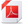## Topic outline

• ### General

•••Book URL
• ### Topic 1. Introduction to Machine learning. History

Cybernetics and the first neural networks
The first digital computers
The emergence of expert systems
Probabilistic approach
Random Forest
Recent achievements

••• ### Topic 2. General concepts

Machine learning problem
Classification and regression
Features and their types
Model and training method. Loss functional
Probabilistic statement of the training problem. The maximum likelihood principle and its relation to empirical risk minimization.  Decision function
Overfitting and the generalizing ability of an algorithm

••• ### Topic 3. Distance-based algorithms

Generalized algorithm
Examples: Nearest Neighbor, Parsen Window method
Margin
The Curse of Dimensionality
Choosing a distance
Distance-based regression

••• ### Topic 4. Bayesian approach

Bayesian approach

Probability density estimation

- nonparametric
- parametric

••• ### Topic 5. Linear classification algorithms

Linear regression
Logistic regression
Margins for a linear classifier
One hot encoding
ROC and AUC
SVM

••• ### Topic 6. Neural Networks

Linear perceptron
Neural nets structure
Backpropagation
Objective functions for regression, binary/multiclass/multilabel classification
Activation functions
Neural nets design

••••• ### Topic 7. Rule-based learning

The concept of rule

The criterion for the rule quality

Finding rules

Classification algorithms based on rules

•